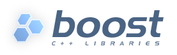Boost.Locale
Text Conversions

There is a set of functions that perform basic string conversion operations: upper, lower and title case conversions, case folding and Unicode normalization. These are to_upper , to_lower, to_title, fold_case and normalize.

All these functions receive an `std::locale` object as parameter or use a global locale by default.

Global locale is used in all examples below.

# Case Handing

For example:

std::string grussen = "grüßEN";
std::cout <<"Upper "<< boost::locale::to_upper(grussen) << std::endl
<<"Lower "<< boost::locale::to_lower(grussen) << std::endl
<<"Title "<< boost::locale::to_title(grussen) << std::endl
<<"Fold "<< boost::locale::fold_case(grussen) << std::endl;

Would print:

```Upper GRÜSSEN
Lower grüßen
Title Grüßen
Fold  grüssen
```

You may notice that there are existing functions `to_upper` and `to_lower` in the Boost.StringAlgo library. The difference is that these function operate over an entire string instead of performing incorrect character-by-character conversions.

For example:

std::wstring grussen = L"grüßen";
std::wcout << boost::algorithm::to_upper_copy(grussen) << " " << boost::locale::to_upper(grussen) << std::endl;

Would give in output:

```GRÜßEN GRÜSSEN
```

Where a letter "ß" was not converted correctly to double-S in first case because of a limitation of `std::ctype` facet.

This is even more problematic in case of UTF-8 encodings where non US-ASCII are not converted at all. For example, this code

std::string grussen = "grüßen";
std::cout << boost::algorithm::to_upper_copy(grussen) << " " << boost::locale::to_upper(grussen) << std::endl;

Would modify ASCII characters only

```GRüßEN GRÜSSEN
```

# Unicode Normalization

Unicode normalization is the process of converting strings to a standard form, suitable for text processing and comparison. For example, character "ü" can be represented by a single code point or a combination of the character "u" and the diaeresis "¨". Normalization is an important part of Unicode text processing.

Unicode defines four normalization forms. Each specific form is selected by a flag passed to normalize function: